Gao, Y., Hua, J., Chen, G., Cai, L., Jia, N., and Zhu, L. (2018). "Prediction of fiber quality using refining parameters in medium-density fiberboard production via the support vector machine algorithm," BioRes. 13(4), 8184-8197.

#### Abstract

Fiber quality greatly influences the performance of medium-density fiberboard (MDF). To evaluate the fiber quality more accurately during refining, a novel quantitative parameter-property relationship model was developed based on the support vector machine (SVM) algorithm. Based on the mill production conditions, a total data set of 1173 experimental fiber quality data points under a wide range of five refining parameters was employed to train and verify the model. By comparing the effectiveness between the model using nonlinear SVM and the model based on multiple linear regression (MLR), the values of mean absolute error (MAE), mean relative error (MRE), root mean square error (RMSE), and Theil’s inequality coefficient (TIC) were reduced 92.19%, 92.36%, 87.29%, and 87.21%, respectively. The results showed that the performance of the predictive model developed using SVM was superior to the MLR model. Furthermore, the variations of the percentage of qualified fibers with each production parameter were predicted using the established model. The prediction model that resulted can be applied to predict the fiber quality during the refining process in an MDF production mill.

Prediction of Fiber Quality Using Refining Parameters in Medium-density Fiberboard Production via the Support Vector Machine Algorithm

Yunbo Gao,a Jun Hua,a,* Guangwei Chen,a Liping Cai,b Na Jia,a and Liangkuan Zhu a

Fiber quality greatly influences the performance of medium-density fiberboard (MDF). To evaluate the fiber quality more accurately during refining, a novel quantitative parameter-property relationship model was developed based on the support vector machine (SVM) algorithm. Based on the mill production conditions, a total data set of 1173 experimental fiber quality data points under a wide range of five refining parameters was employed to train and verify the model. By comparing the effectiveness between the model using nonlinear SVM and the model based on multiple linear regression (MLR), the values of mean absolute error (MAE), mean relative error (MRE), root mean square error (RMSE), and Theil’s inequality coefficient (TIC) were reduced 92.19%, 92.36%, 87.29%, and 87.21%, respectively. The results showed that the performance of the predictive model developed using SVM was superior to the MLR model. Furthermore, the variations of the percentage of qualified fibers with each production parameter were predicted using the established model. The prediction model that resulted can be applied to predict the fiber quality during the refining process in an MDF production mill.

Keywords: Fiber quality; MDF; Refining; Predictive model; SVM

Contact information: a: College of Electromechanical Engineering, Northeast Forestry University, Harbin, 150040, China; b: Mechanical and Energy Engineering Department, University of North Texas, Denton, TX 76201, USA; *Corresponding author: huajun81@163.com

INTRODUCTION

Medium-density fiberboard (MDF) has been widely applied in the furniture and interior decoration markets due to its dimensional stability, workability, flatness, smooth appearance, good bond strength, and screw-holding ability (Hua et al. 2012). A crucial step in the fiberboard production is the refining process (Runkler et al. 2003). It is impractical and costly to evaluate which fiber qualities are affected by certain production parameters through experimental determination techniques during the refining process. Production parameters are mainly adjusted based on the experience of workers, which suffers from poor accuracy due to the lack of theoretical guidance on the model of fiber quality relating to production parameters. Therefore, it is essential to develop new models to predict fiber quality according to production parameters.

To investigate the influence of production parameters during refining on the fiber or the fiberboard quality, some studies have been carried out over the past decade. Chen and Hua (2009) developed a constraint relationship between the fiber productivity and fiber quality using third-order polynomial and linear regressions, and an optimization method was developed to adjust the fiber quality by modifying fiber productivity that depends on the feeding screw revolution speed and the opening percentage of the discharge valve. The relationship between the content of bark and the fiber quality was investigated by Jia et al. (2015). It was demonstrated that the fiber screening value first presented an increasing trend and then descended with an increase in bark content. Xing et al. (2006) investigated the influence of thermo-mechanical reﬁning on the properties of MDF panels made from black spruce bark, and the results showed that the preheating retention time was an important factor for both the modulus of rupture and the modulus of elasticity. The steam pressure was an important factor for internal bond strength, modulus of rupture (MOR), and modulus of elasticity (MOE). The previously described studies revealed the relationship between the production parameters during refining and the quality of fiber or fiberboard based on linear or polynomial regression, which was shown to be disadvantaged by low accuracy and the inability to predict the quality online.

To address these drawbacks, some researchers developed models between the production parameters during refining and the quality of fiber or fiberboard with intelligent algorithms. To determine the process set points leading to minimum production cost for the target quality, Gerstorfer et al. (2001) established a Takagi-Sugeno-Fuzzy model for the refining process based on the experts’ knowledge as well as the data collected. The neuro-fuzzy modeling methods were used by Runkler et al. (2003) to model the wood chip reﬁner process for ﬁberboard production to provide online predictions of the two important quality indices (ﬂexural strength and water uptake). The results showed that the model accuracies achieved were approximately ± 5 N/mm2 for ﬂexural strength and approximately ± 10%/24 h for water uptake. However, the fuzzy rules were determined on the dependence of experts’ technological experience, which only leads to a certain extent of improvement on the prediction accuracy. Because the artificial neural network (ANN) can model highly nonlinear systems without using complex deduction rules or large data (Huang and Lu 2016), it was used as a predictive method to determine the moisture resistance of particle- and fiberboards under cyclic testing conditions by Esteban et al. (2010). However, the training procedure for ANN models is not only time consuming but it is also possible to get trapped in local minima (Hong et al. 2013).

With the advantages of a simple structure, good generalization ability, nonlinear modeling properties (Wang et al. 2009; Zhou et al. 2016; Sun et al. 2016), and avoidance of the problems of over-ﬁtting, local extremum, and dimension disaster (Shi et al. 2010; Zhao et al. 2014; Sun et al. 2016), the support vector machine (SVM) has become a promising classification and regression algorithm. The SVM can be used for classification of data and text, system modeling and prediction, pattern recognition, anomaly detection, and time series prediction (Jiao et al. 2016) in many fields (Mokhtarzad et al. 2017; Roushangar and Ghasempour 2017; Huang et al. 2018). Among these fields, Zhang et al. (2016) employed the SVM method to establish parameters-properties models in the papermaking field. Although SVM has been employed in many fields because of its advantages of nonlinear relationship expression, it has not been used for the modeling of fiber quality during the refining process in MDF production.

This study is aimed at increasing the percentage of qualified fibers (QF) by adjusting five parameters, i.e., the conveyer screw revolution speed (SR), accumulated chip height (CH), opening ratio of the discharge valve (OV), content of log bark (CB), and content of Chinese poplar (CP) during refining. The QF were evaluated by fiber size, i.e., the screen mesh grades of the fibers. Firstly, a large amount of fiber quality data was collected from the MDF production mill under a wide range of SR, CH, OV, CB, and CP to uncover the relationship between the refining parameters and fiber quality. Secondly, SVM was utilized to construct the nonlinear predictive model of fiber quality during the refining. The experimental values and predicted outputs of the model were compared, and the accuracy of the model was established. Thirdly, the results from the SVM-based model and the multiple linear regression (MLR)-based model were compared, showing that the SVM model predicted fiber quality more effectively and accurately than that from the MLR.

EXPERIMENTAL

Materials

Data collection

The data were collected in a production line at a MDF mill in Northern China and two major species, namely Chinese poplar (Populus lasiocarpa Oliv.) and Chinese larch (Larix potaninii Batalin), were used in the production. In the production line, the 4000 kW refiner (model: 50-ICP; Andritz Group, Graz, Austria) utilized had double 1372-mm diameter disks running at a rotational velocity of 1,500 r/min.

Figure 1 shows the major components of the refiner. A feeding screw (2) transfers the wood chips in the hopper (1) to the pre-heater (3). The preheating retention time was determined by CH. Chips were discharged to the refiner (6) using a conveyer screw (4) after the steam-softening. Through the discharge pipe (5), the refined fibers were unloaded under the steam pressure in the refiner. The opening ratio of the valve installed on the discharge pipe (5) was used to adjust the amount of unloaded fibers.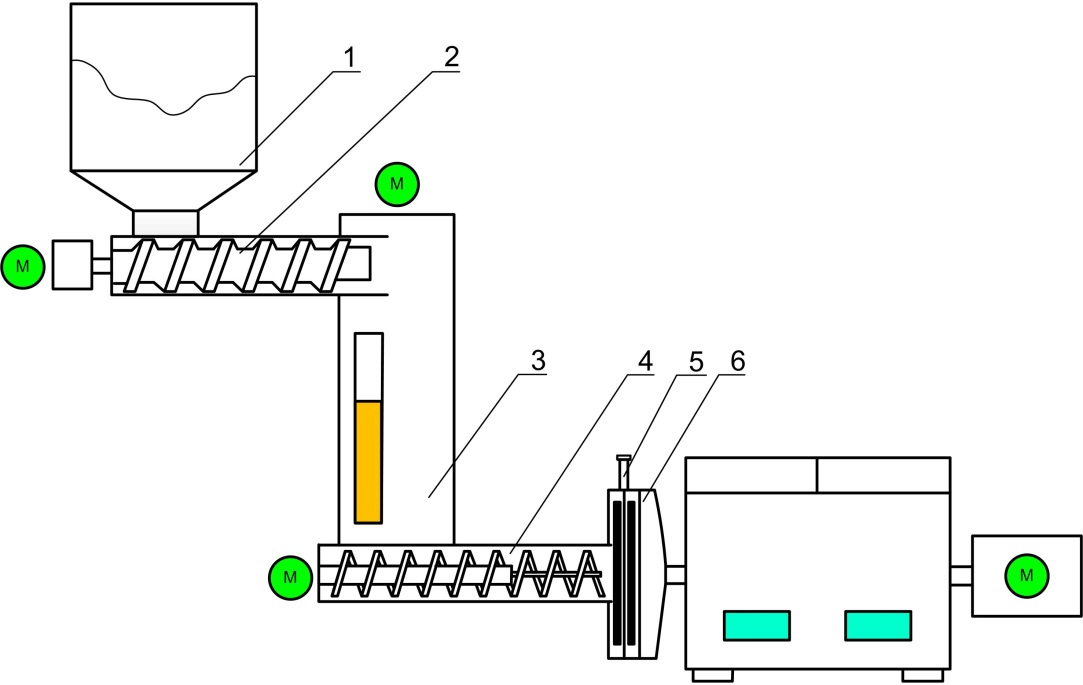Fig. 1. The major components of the refiner: 1) Hopper, 2) Feeding screw, 3) Pre-heater, 4) Conveyer screw, 5) Discharge pipe, and 6) Refiner

In the chip washing and steaming processes, the moisture content of the wood chips increased. However, during the chip transportation, the feeding screw squeezed the moisture from the chips in the feeding pipe and brought the final moisture content of the chips to 50%.

In the pre-heating process, the steaming pressures usually slightly fluctuate from 0.766 MPa to 0.990 MPa (corresponding to a steaming temperature that ranged from 168.6 °C to 179.5 °C). The gap between the two refining disks was pre-set to 0.1 mm. The sensors installed on the production line measured five refining parameters, including SR, CH, OV, CB, and CP, hourly under usual production conditions.

Generally, large fiber size would produce panels with poor board appearance, while smaller-sized fibers could cause the reduction in panel strength (Shi et al. 2006). Technically, the good fiber shape for MDF requires a moderate ratio of length/width of the fiber (Chen 2012). Based on the mill’s practices, the fibers with a size between 20 to 120 screen mesh were considered to be qualified in this study.

In the fiber processing, 10 g of fiber were collected and weighed with a balance off the production line to determine the fiber sizes for each measurement. The percentage of the QF in the total amount = (The weight of qualified fiber (g) / 10 g) × 100%.

It was hypothesized that the response variable (QF) during the refining could be estimated by correlating the predictor variables (SR, CH, OV, CB, and CP). A large sample size, 1,173 measurements for each variable, was analyzed in this study. Two models, namely, MLR and SVM, were developed for predicting the fiber quality. The accuracies of the two models were validated and compared using the data collected in the MDF production line.

Methods

Support vector machine algorithm

The SVM, proposed by Vapnik (1999), is a relatively new and promising classification and regression algorithm based on the statistical learning theory and structural risk minimization principle. Based on this principle, SVM possesses an optimum network structure that is beneficial to reduce the global error of the model (Xiao et al. 2014).

The basic principle is as follows (Chu et al. 2017). Training data are presented in the form{xiyi}n i=1, where xi values are the input data, yi values are the corresponding output data, and nis the number of training data points. The SVM is used to search an optimal regression function, which can estimate all of the training data (Drucker et al. 1997). The regression function can be expressed as,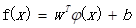(1)

where wRn denotes the weight vector, φ(x) denotes the nonlinear mapping function, and b denotes the bias. As mentioned previously, SVM is based on risk minimization, while w and bare estimated by minimizing the regularized risk function as below,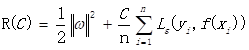(2)

where 1/2‖w‖2 is the flatness of the function, C is the penalty factor, which is the correlation between empirical error and flatness of the model (Yan and Shi 2010), ε is a prescribed parameter, and Lε(yif(xi)) is the ε-insensitive loss function that can be defined as: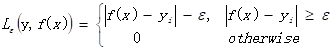(3)

By introducing the slack variables ξ and ξ*, Eq. 2 can be written as: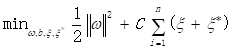(4)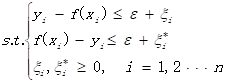(5)

The dual objective optimization problem can be solved using the Lagrange multipliers (Ma et al. 2003). Finally, the regression function is obtained as the following equation,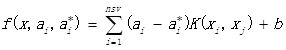(6)

where aand a* i are the Lagrangian operators, nsv is the number of support vectors, and K(xiyi) is the kernel function. It is important to select the kernel function and its parameters because the generalization performance of SVM depends on the type of kernel function, its parameters, and several internal parameters of SVM (Zhao et al. 2016).

The Gaussian Radial Basis Function (RBF) is mostly used for the kernel function due to its properties of good generalization and nonlinear forecast as well as its characteristic of few parameters that need to be adjusted (Bishop 1995; Keerthi and Lin 2003). Therefore, in this study, the Gaussian RBF was selected as the kernel function using the following formula,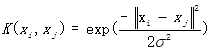(7)

where σ is the width of RBF.

Therefore, there are two variables that need to be selected in the SVM model, which are the constant “C” and the width of the Gaussian RBF kernel “σ”. In this study, the optimization of these parameters was performed by a systematic grid search of the parameters using the cross-validation on the training set.

The SVM predictive model for fiber quality

In this paper, the software used for analysis was Matlab (MathWorks, R2010a, Natick, MA, USA). The diagram of the SVM predictive model is illustrated in Fig. 2. The details of the operation process for the SVM model are discussed below.

Step 1: Data preprocessing

To ensure the training stability of the SVM and avoid the bad influence caused by discrepancy of quantitative dimension, the data from experiments were normalized with the following mapping function,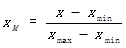(8)

where xis the normalized data, x is the original data, and xmax and xmin denote the maximum and minimum raw input values, respectively. The original data were normalized to the range of 0 to 1.

Step 2: Cross validation (CV) to select the best parameters of regression, C, and σ

First, the fitness function (mean square error) was determined based on 3-CV, ε was pre-set as 10-4, and the range of C and σ were defined and meshed. Second, the fitness function was recalculated through updating C and σ within the grid. Finally, the C and σ that generate the minimum average mean square error (MSE) of the three models were selected as the best parameters.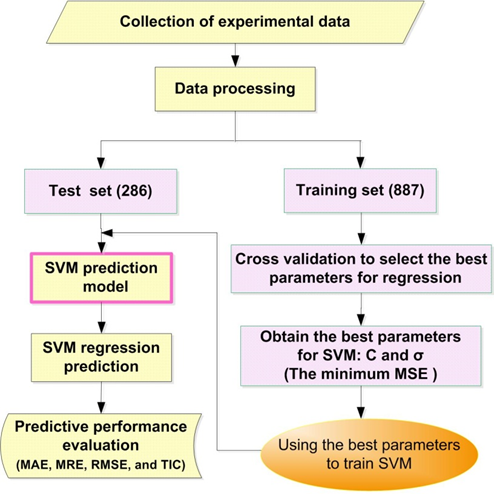Fig. 2. Diagram of the support vector machine predictive model

Step 3: Building the SVM model for fiber quality

The SVM model for fiber quality was trained and established based on the best parameters obtained in step 2, which can be used to investigate the relationships between the parameters and the property.

Step 4: SVM regression prediction

The built SVM model was used to predict the training set and test set, and then the fiber quality under prediction was compared with the experimental data.

Step 5: Evaluation of predictive performance

The predicted performance was evaluated in terms of mean absolute error (MAE), mean relative error (MRE), root mean square error (RMSE), and Theil’s inequality coefficient (TIC). They are defined according to the following formulas, respectively,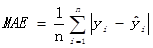(9)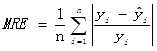(10)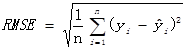(11)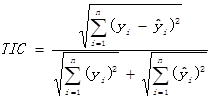(12)

where yi are the actual outputs (experimental qualified fibers),the outputs of models (predicted qualified fibers), and n is the number of compounds in the analyzed data set.

RESULTS AND DISCUSSION

Results of the SVM Algorithm

The inputs for the fiber quality model using the SVM algorithm were SR, CH, OV, CB, and CP, and the output was QF. Out of a total set consisting of 1173 groups of data from the experiment, 887 groups of data were used to build the model as a training set, while 286 groups of data were used to validate the model established as a test set. In selecting the SVM parameters, K-CV can effectively avoid the occurrence of overlearning and under-learning. In this paper, the optimization of the SVM parameters was carried out by 3-CV. Figure 3 illustrates the SVM parameter selection process and results (contour map and 3D view). As shown in Fig. 3a, contour lines represent the C and σ corresponded MSE based on the K-CV method. The most optimum condition where the MSE of the 3-CV method equaled 0.00025373 was selected. The final optimization results were C = 1 and σ = 5.6569.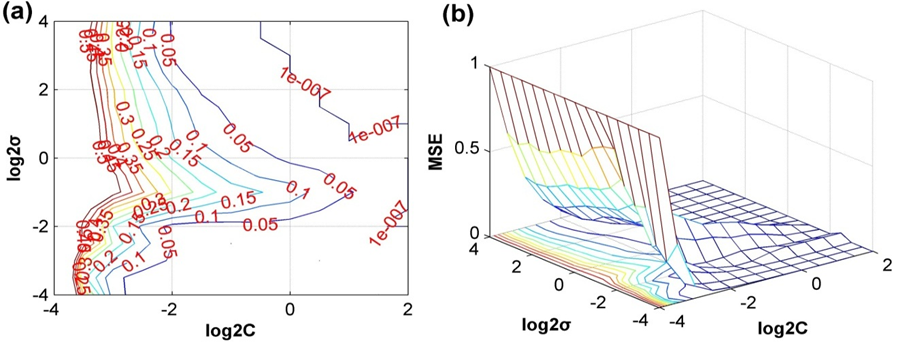Fig. 3. SVM parameter selection process and results: (a) contour map, and (b) 3D view

To estimate the accuracy of the fiber quality model, the experimental data were compared with the predicted outputs as shown in Fig. 4. In Fig. 4a, the scatter plots of the predictive versusthe experimental outputs for the qualified fibers were observed. If the model exactly matched the actual values, all data points would be on the main diagonal. It was revealed that the data points were indeed close to the main diagonal, indicating good model accuracy. As described in Fig. 4b, the relative deviations of the predicted qualified fibers values in comparison with experimental values indicated that the new model established by the SVM algorithm clearly possessed promising prediction properties.